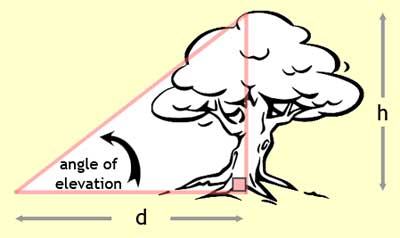Trigonometry Word Problem Quizmaster
Review the Lesson | MATHguide homepage Updated July 21st, 2021

Waiting for your answers to the problem below.

Answer the following trigonometry problem. Report the answer to the nearest tenth.

 A forest ranger wanted to calculate the height, h, of a large tree. He walked 96 yards from the base of the tree. [See the distance, d, in the diagram.] From that location, he measured the angle of elevation to the top of the tree and it was 40°. What is the height of the tree?h = yards